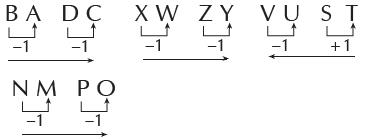Courses

# Olympiad Test: Classification - 1

## 15 Questions MCQ Test Science Olympiad for Class 5 | Olympiad Test: Classification - 1

Description
This mock test of Olympiad Test: Classification - 1 for Class 5 helps you for every Class 5 entrance exam. This contains 15 Multiple Choice Questions for Class 5 Olympiad Test: Classification - 1 (mcq) to study with solutions a complete question bank. The solved questions answers in this Olympiad Test: Classification - 1 quiz give you a good mix of easy questions and tough questions. Class 5 students definitely take this Olympiad Test: Classification - 1 exercise for a better result in the exam. You can find other Olympiad Test: Classification - 1 extra questions, long questions & short questions for Class 5 on EduRev as well by searching above.
QUESTION: 1

### In the following questions, three out of four options given below are alike in a certain way and so form a group. Which is the one that does not belong to the group?

Solution:

Except (B), all are items of stationery

QUESTION: 2

### Three of the following four options are alike in a certain way and form agroup. Which is the one that does not belong to the group?

Solution:

Except (B), all are fruits. Jackfruit is a
type of vegetable.

QUESTION: 3

### Three of the following four options are alike in a certain way and form a group. Which is the one that does not belong to the group?

Solution:

Except (A), all have engines.

QUESTION: 4

Three of the following four options are alike in a certain way and form a group. Which is the one that does not belong to the group?

Solution:

Except (A), all are parts of a tree.

QUESTION: 5

Three of the following four optionsare alike in a certain way and form a group. Which is the one that does not belong to the group?

Solution:

Except (C), all are names of birds.

QUESTION: 6

Three of the following four options are alike in a certain way and form a group. Which is the one that does not belong to the group?

Solution:

Except (D), all are involved in purchasing
and selling.

QUESTION: 7

Three of the following four options are alike in a certain way and form agroup. Which is the one that does not belong to the group?

Solution:

Except (C), all are pieces of furniture.

QUESTION: 8

Three of the following four options are alike in a certain way and so form a group. Which is the one that does not belong to the group?

Solution:

Except (A), all are found in pairs in
our body.

QUESTION: 9

Three of the following four options are alike in a certain way, and form a group. Which is the the one that does not belong to the group?

Solution:

Except (D), all are units of mass.

QUESTION: 10

Three of the following four options are alike in a certain way and form a group. Which is the one that does not belong to the group?

Solution:

Except (C), all are science subjects.

QUESTION: 11

Three of the following four options are alike in a certain way and options form a group. Which is the one that does not belong to the group?

Solution:

Except (A), all are caused by virus
Rickets is a deficiency disease.

QUESTION: 12

Three of the following four options are alike in a certain way and form a group. Which is the one that does not belong to the group?

Solution:

Except (B), all colours are found in
rainbow.

QUESTION: 13

Three of following four options are alike in a certain way and form a group. Which is the one that does not belong to the group?

Solution:

Except (B), all others are drink.

QUESTION: 14

Three of following four options are alike in a certain way and form a group. Which is the one that does not belong to the group?

Solution:

Except (C), all others are capital cities
of countries.

QUESTION: 15

Three of the following four are alike in a certain way so form a group select the one which does not belong to the group.

Solution:

Except (C), all have definite order.Track your progress, build streaks, highlight & save important lessons and more!

### Similar Content### Related tests